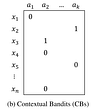# RL — Reinforcement Learning Algorithms Comparison

Choosing an RL algorithm can be confusing. In this article, we will focus on different decision factors in choosing your algorithms.

• Sample efficiency — how many samples are needed to train a good policy?
• Stability & convergence — how easy & how fast the model converges.
• Generalization — Will the model generalize to other tasks?
• Assumptions & approximation — Does the method have any other constraints? Does the method work on discrete or continuous action space? What approximate is used?
• Exploration — How well does it explore the action space?
• Policy-centric v.s. Model-centric.

What are expensive?

• Real-life simulation is expensive. Physical simulation is 10,000 times slower than computer simulation like MuJoCo. But for robotic controls, real-life simulation seems unavoidable.
• Many problem domains have steep curvature for their reward function. This leads to unstable training that destroys training progress easily.
• Large-scale problems need high parallelism to speed up the calculations. Some RL methods like value-based learning and model-based RL are not obvious on how to parallelize works.
• The iterative methods in Model-based solutions are computationally expensive.

# Bias v.s. Variance

Increasing sample efficiency, lower bias, and lower variance are often conflicting objectives. In machine learning, errors are composed of bias and variance. One method can reduce variance but increase bias badly, or vice versa. When making design choices, we often juggle between these tradeoffs.

Monte-Carlo methods have high variance but zero bias. A stochastic policy produces different trajectories in different runs. In RL, tiny changes in the trajectory can lead to different rewards. Hence, MC has a high variance over different runs.

On the other hand, 1-step Temporal Difference learning uses a single-step lookup in computing the value function. Since only one action is involved, the change is small and the variance is low. But the result is biased, in particular during early training.

Policy Gradient (PG) is vulnerable to variance. One calculation may tell us to increase the chance of an action while another tells us to decrease it. It hurts model convergence. There are many methods to reduce its variance. We can use a better advantage function or restrict the gradient changes with trust region, etc...

Another way of reducing variance is to increase the number of sampling. Run MC many times to find the average or use a large batch size for each training iteration. But this hurts sample efficiency. When simulations take time, low sample efficiency hurts.

Off-policy actor-critic, Q-learning, and many value-fitting methods adapt the TD concepts. While they have lower variance, they often require extensive hyperparameter search to make it works. The hypersensitive in hyperparameter tuning hurt stability and generalization.

# On-policy learning v.s. Off-policy learning

PG methods sample actions using a policy 𝜋. Then we observe the rewards to optimize 𝜋. In short, we make high-reward actions more likely using gradient ascent. For value-learning methods, we use the observed rewards to fit a Q-value function instead and use the Q-value function to derive a policy. Regardless of the difference, the policy to sample data and the policy to be optimized is the same policy 𝜋. This is called on-policy as there is only one policy throughout the iterative process.

In the on-policy example below, we sample and optimize an ε-greedy policy 𝜋. As a side note, 𝜋 is a sub-optimal policy and this is a hack to improve exploration. However, after the training, we can derive the optimal policy from Q.

On-policy methods are usually simpler, less variance, and faster to converge compared with off-policy.

In the example above, we introduce hacks to improve its shortcomings. But these issues can be addressed in a more flexible way by having two separate policies: a behavior policy for us to generate samples and a target policy for us to optimize.

For on-policy methods, behavior policy and target policy are the same. But in off-policy methods, they are not. These policies may be loosely related or even unrelated. For example, the behavior policy can be a random policy. This is completely unrelated to 𝜋 to improve exploration, even though this will be an overkill.

The off-policy methods are designed to address different shortcomings with tradeoffs in simplicity and convergence. For example, some off-policy methods improve exploration and some methods improve the target policy without generating new samples constantly.

In on-policy methods, samples are generated from a policy that is actively optimizing. Changes in policy may require new samples to improve it. This hurts sampling efficiency badly. As shown below, the gradients of PG methods are computed using samples collected from 𝜋. If 𝜋 is changed, we should draw new samples again. This is awfully inefficient when a trajectory may contain hundreds of moves and the samples collected are good for a single gradient update only.

To improve sample efficiency, off-policy learning can use an additional policy to give explicit control over what samples to use in optimizing the target policy. Specifically, many on-policy methods can be modified to become off-policy methods using importance sampling (1, 2). We use a slightly staled policy θ’ as the behavior policy so old samples can be reused. We recalibrated the old observed rewards with importance sampling to approximate the rewards under the target policy θ distribution. These recalibrated rewards will be used for gradient descent to optimize the target policy θ. We synchronize θ’ with θ regularly, say every four updates, to improve the accuracy of our estimation.

DQN is another off-policy method. We draw samples from a replay buffer to fit the Q-value.

Q-learning is off-policy. It uses ε-greedy to sample actions. Q function is then fitted for an optimal policy.

For on-policy methods, there is only one policy while off-policy methods have more than one. As shown below, SARSA has only one policy (an on-policy method) while Q-learning (a.k.a. SARSA max) has two policies.

Off-policy methods usually take advantage of the second policy to improve sample efficiency. However, for some RL methods, many people find it hard to determine whether they are on-policy or off-policy. Instead of what labels are given to an algorithm, we can just focus on its specific design — what it improves or sacrifices. For Q-learning, A’ is chosen by the target policy derived by Q. This helps stability as the choice of A’ is less random compared with ε-greedy. In DQN, the replay buffer improves both stability and sample efficiency. For other designs, the key focus may be on exploration.

# Sample efficiency

Evolutionary algorithms, a type of educated random guessing algorithm, have the lowest sample efficiency. Full online methods (like A3C) sample data and update policy iteratively. The sampling efficiency improves by 10x. Here are the levels of efficiency compared with other RL methods.

On the other extreme, model-based methods take advantage of the inner understanding of the system dynamics. This knowledge reduces the volume of the samples required for training. But to feed a deep network inside model-based methods, we need 10 times more samples compared with a shallow model. Policy Gradient (PG) methods heavily depend on sampling to estimate rewards. The less we know about the model dynamic, the more trial and error is needed to know what policy works.

On-policy methods update and sample from the same policy. Changes in the policy need new samples to optimize it. By separating the target policy from the behavior policy, we are given a chance to reuse samples. Vanilla PG method, an on-policy, has a poor sample efficiency. Q-learning, utilizing off-policy, is designed to improve sample efficiency during model fitting.

Here is the plot on the performance of many model-free methods. As noted, it takes easily 80+ million frames for many advanced methods to outperform the human expert in playing Atari games. The graph below is normalized. An average human expert scores at 100% below.

Low sample efficiency makes many real-world problems impractical to solve. For future research, we may find better model-based algorithms, faster algorithms and apply accumulated knowledge (prior knowledge) in solving problems.

# Sample efficiency v.s. Wall clock time

Sample efficiency is not necessarily an ultimate goal. There is no free lunch. If you have fewer samples, you need to achieve better optimization per sample. Model-based methods are most sample efficient but have the most complex optimization calculation. When sampling is cheap or can be done efficiently with simulation, model-free methods may achieve shorter wall clock time in training.

For robotic control, collecting samples are expensive compared to the optimization calculation. The trajectory planning is just a small part of the wall clock time in a model-based method. Therefore, it can favor model-based methods more.

In addition, as the amount of samples increase, data parallelism becomes viable and changes the landscape of which methods are better. The Evolutionary methods use some kind of “educated” random guessing on the model parameters to solve problems. It has very low sample efficiency. But since it has low computation usually and it is highly parallel, it becomes a feasible solution for many problems.

Model-free RL methods are more appealing when collecting samples are cheap.

# Stability & Convergence

Gradient descent is heavily studied with nice convergence if the objective function is convex. However, many RL methods are not based on gradient descent. Therefore, new questions are raised on the convergence of RL methods. We need to ask:

• whether the solution converges,
• where it converges, and
• how often it converges (how sensitive to random seeds and hyperparameters)

Value fitting algorithms have no guarantee of convergence if a nonlinear approximator, like a deep network, is used to fit the value function. Indeed, convergence is most problematic for value-learning algorithms comparing with Policy Gradient and model-based methods. Unlike deep learning, samples collected in a trajectory is highly related. Gradient changes are magnified un-proportionally resulting in training instabilities. In addition, training creates moving target values. It is hard to train a deep network steadily if the target value keeps changing.

Model-based methods fit the system dynamics. These methods usually converge. However, the solution is usually less generalized. When dealing with situations that are unfamiliar, the prediction can be erroneous. The stability will depend on many factors including what model class (like Gaussian Process, GMM), global or local model, how many steps to predict, model outputs (like a Gaussian distribution), and the algorithm in fitting the model (details on model-based methods). If a policy is optimized using backpropagation through time from the model (like PILCO), the process is usually non-trivial. If we learn a policy with deep networks using samples from the model, the process will inherit problems in model-free methods. And if the model is flawed, the policy may exploit the model for maximum rewards that result in unrealistic behaviors.

The gradient descent in Policy gradient methods will converge for convex objective functions. But Policy Gradient has high variance and bad sample efficiency. We can make bad decisions that destroy the training progress. We can use a baseline function or a large batch size to address the problem. A large batch size hurts the sample efficiency further but it usually generates better results. Designing a better baseline to reduce variance is important but it will take a lot of engineering efforts. Tuning the learning rate or schedule in PG is particularly challenging. Other key hyperparameters to be tuned is the batch size, and the baseline.

Researchers are actively improving algorithms for stability. And we can shop for algorithm enhancements to lessen these problems. For PG methods, one possibility is to introduce trust-region concepts like TRPO, and PPO.

For value-learning, they are addressed by experience replay and target network in DQN. Nevertheless, these improvements in value-learning introduce many hyperparameters including target network delay, replay buffer size, clipping, and learning rate schedule, etc… Hyperparameter tuning for value-fitting algorithms is considered to be harder. In practice, with patient tuning, the model can converge. But these hyperparameters may not generalize well to other RL tasks. Another round of major tuning may be required.

Many algorithms can run into bad local optima. We can address the problem by training different models with different random seeds. Then, we use the one with the best-expected reward.

The choice of algorithms can be task-sensitive as they have different curvature characteristics in their reward function. Therefore, some experiments are needed in selecting the algorithms.

More researches may be needed to develop methods in adaptive parameters during training (for example, the adaptive control variate/baseline in Q-prop.), reducing hyperparameters, automatic hyperparameter tuning and less hyperparameter sensitivity.

# Hyperparameters

Tuning hyperparameters in RL is even harder than deep learning. We can use software simulators instead of real-world experiments to do the first-round hyperparameter searches with the hope that these hyperparameters are good enough as a first start. We can also look into methods like evolutionary methods or AutoML to search the hyperparameter space better.

# Assumptions & Approximation

Many RL methods make assumptions. The following lists some assumptions that we need to be aware of.

• Continuity: In value fitting methods and some model-based methods, we assume the action space and/or the state space is continuous. This is one assumption that needs to be verified before choosing any RL methods.
• Discrete or continuous control space: DQN is mainly for low-dimensional discrete control space. Q-learning is a SARSA max method. In step ③ below, it requires complex optimization to locate max Q(s, a) for continuous control space. This is often too complex to be practical. Policy gradient methods, including the Actor in the Actor-critic method, support both discrete and continuous control. One exception is the DDPG which supports continuous control space only.
• Full observability: Views may be blocked temporarily. It hurts the value-learning methods that depend on the current state. Nevertheless, we can concatenate consecutive states together or use RNN to remember historical information.
• Episodic learning: In some policy gradients or Model-based RL methods, we assume the environment can be reset to start another episode.
• Stochastic or deterministic dynamics/policy: Most RL methods make assumptions that the dynamics or the policy are stochastic.

Approximations are often made to reduce the memory footprint or to reduce the computational complexity. In the former case, a function approximator, like a deep network, is often used to cover all possible discrete space (states or actions) or a continuous space.

# Exploration

Off-policy learning has an additional policy that gives explicit controls over exploration. For example, Q-learning uses ε-greedy exploration to pick the first action with the objective of picking something promising but yet giving chance to actions that are less studied.

Off-policy learning has more freedom to explore than on-policy. This exploration can build a broader understanding of the domain in finding a better solution. But it is at the cost of training efficiency. However, for many robotic tasks, our objective is getting things done rather than finding the perfect way to do it.

But when AlphaGO defeated the GO champions, one comment from the champion is half the moves from AlphaGO is unexpected. A border exploration may be key to beat humans. Human is not prepared for these scenarios and less likely to give an optimal response.

# Model-free v.s. Model-based RL

Model-free RL focuses on sampling to build up a policy or value functions. It ignores the model dynamics completely. Model-based methods use the model to find the optimal policy. It does not need to create a policy explicitly.

Some model-based methods have explicit assumptions to make optimization easier. For example, the cost function may be approximated to be quadratic, and the dynamics be locally linear. Model-free RL emphasizes learning from mass samples with fewer assumptions on the system dynamics or the reward functions. It can apply to a wider spectrum of tasks and is less specialized than the model-based RL methods. Let’s summarize the key difference here.

Model-based methods

Strength:

• It can be self-trained and therefore more scaleable.
• Sample efficient.
• The learned dynamic model is transferable.

Weakness:

• But need retraining to optimize the controller again for a specific task.
• Not optimizing the policy directly.
• More assumptions and does not work with all tasks.
• A model can be much complex to train than a policy.

Model-free methods

Strength:

• It has fewer approximations and assumptions that work with a wider spectrum of tasks.
• Good at learning complex policies.
• The policy can be generalized better in some tasks.

Weakness:

• Less sample efficient.
• Vulnerable to overfit with a complex model. Lead to poor decisions.

# Model-centric v.s. Policy-centric

Is a task easier to be modeled by the policy or the model?

In the cart-pole problem, when the pole falls to the left, we move left. We can balance the pole without any Physics equations. Policy learning can be more intuitive and potential simpler for some tasks. The principle discovered by the policy can be very basic and generalize well to other tasks. If we want to understand the dynamic model, we may need more head-scratching.

But finding a model is not always hard. In GO, the model is the rule of the game. We can use it to search for promising moves. On the contrary, it is not easy to know what to do from looking at a board for a beginner. Modeling such a policy directly seems harder.

As discussed before, the smoothness of those functions may play a major role also. As a nice rule, we may ask from the human perspective, is the policy or the model may look simpler? But as a reminder, they are not mutually exclusive. many methods combine both model-based and model-free methods. Even when we start from a model-based method, if a policy is relatively easy to learn, we can use the model to create training samples for the policy to learn through supervised learning. The trained policy may be more generalized than a dynamic model for situations that have not been closely trained.

In Q-learning, we need to find the maximum Q-value for the next action.

However, maximizing Q-value is difficult for a continuous control space.

We can solve it as an optimization problem by searching the control space for the maximum Q-value. But such optimization is computationally intense.

For Policy Gradients, it is easy to set up. The policy is easy to interpret. It optimizes the policy directly. Training a CNN or RNN using the Policy Gradient end-to-end is simple and we can add any constraints or incentives into the objective functions. Nevertheless, the sample efficiency and high variance is a major issue. We can use TD, a baseline, a larger batch size, or a critic to reduce variance. Nevertheless, a larger batch size hurts sample efficiency, and TD & the critic method increase bias.

For value learning, the process is less obvious and less interpretable. Initialize a policy imitating a demonstration is much easier than initiating a value function. However, value learning is more sample efficient. Value-learning methods can use off-policy learning and experience replay to boost sample efficiency.

Policy-based methods optimize a policy directly. Other methods may develop a dynamic model or fit a value function to create an implicit policy after the training. Assumptions and approximations introduced by those methods may cause inefficiency in between. In short, minimizing the fitting error or creating the best model is not exactly the same as optimizing a policy directly. But it explores less compared with other methods.

# Recap

Here is a recap of what we have discussed:

# Select RL algorithms

What is the best RL algorithm? I don’t think we know the answer yet. We make constant advancements in different approaches and we may mix different methods to reduce the bias and the variance.

However, computation simulation in MoJoCo is 10,000 faster than the physical simulation. Sample efficiency often stands out as the most important factor to choose the primary method. Below is a rough guideline if that is the case.

As the primary method is chosen, we may supplement it with other approaches if necessary. For example, we can add policy learning to the model-based RL (GPS) or value-learning to the Policy gradient.

# Demonstration

Value-learning: DQN

Q-learning

Model-based RL

# Credit and references

UC Berkeley Reinforcement learning course

--

--

--

## More from Jonathan Hui

Deep Learning

Love podcasts or audiobooks? Learn on the go with our new app.

## Scratch to SOTA: Build Famous Classification Nets 4 (GoogLeNet)## Using Tensorboard in Google Colab with PyTorch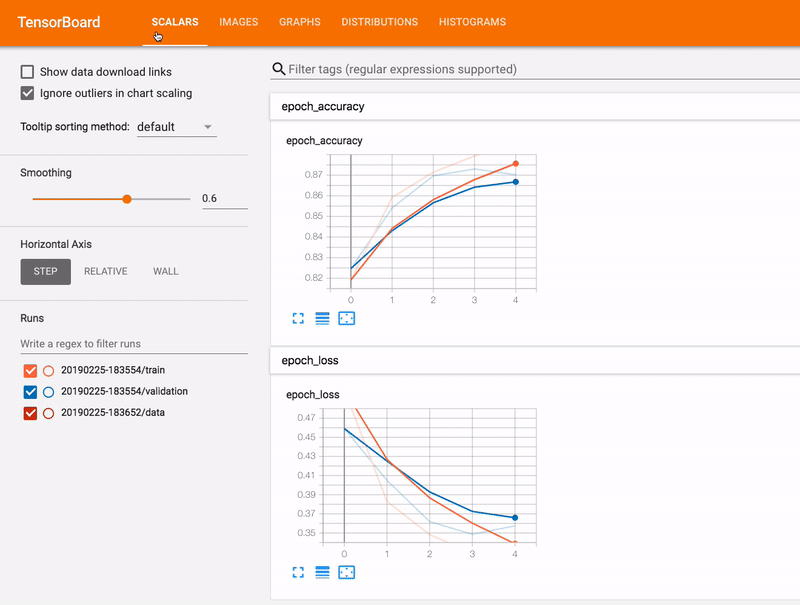## What are all these terms … Data Science, Machine Learning, Artificial Intelligence? | AI MakerSpace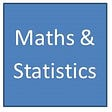## How to save \$340 on a single ML experiment using RAPIDS?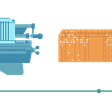## Reinforcement Learning without Reward Engineering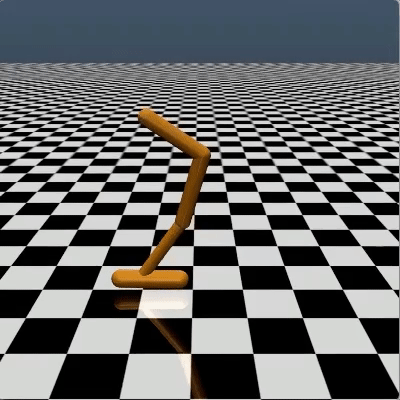## Machine Learning in 10 minutes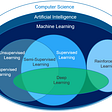## RL — Dual Gradient Descent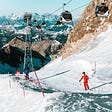## Random Walk in Node Embeddings (DeepWalk, node2vec, LINE, and GraphSAGE)Deep Learning

## Review of Reinforcement Learning Papers #13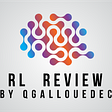## Interaction-Grounded Learning: Learning from feedback, not rewards